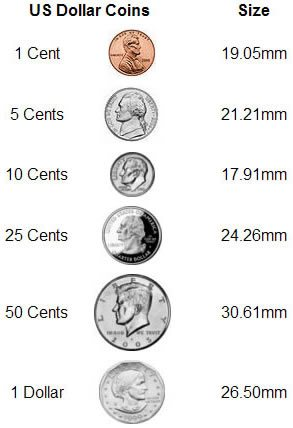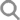登录 注册 只提供英语客户支持 1-800-464-1640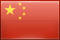USD 宝石 宝石按颜色 宝石按颜色 人气宝石 人气宝石 新品 宝石价格 成对宝石 集合石 标准化 诞生石 诞生石
Jun 01, 2022 更新 Jun 04, 2023

# 来自赞比亚的祖母绿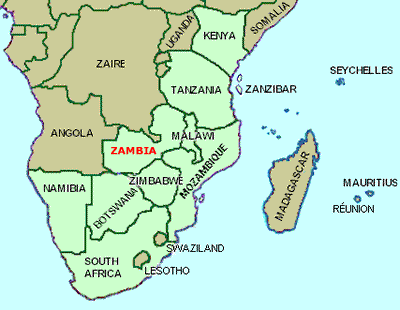南部非洲地图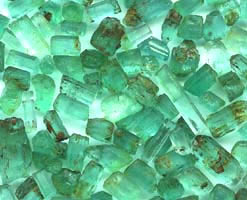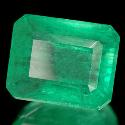Kagem 是赞比亚最大的祖母绿矿，生产高品质祖母绿的历史悠久。在过去的三年中，Kagem 的平均产量为 650 万克拉每年的祖母绿。

 保持联系 *您正在注册接收 GemSelect 促销电子邮件.

15140

 更多形状

•  蓝宝石
•  祖母绿
•  红宝石
•  海蓝宝
•  锆石
•  蛋白石
•  黄玉
•  碧玺
•  石榴石
•  紫水晶
•  黄水晶
•  坦桑黝帘石

•  Nuummite
•  七彩电镀水晶
•  中长石拉长
•  光谱仪
•  卢比莱碧玺
•  变色宝石
•  变色水铝石
•  变色石榴石
•  变色萤石
•  坦桑黝帘石
•  堇青石
•  天河石
•  孔雀石
•  尖晶石
•  巧克力蛋白石
•  帝王黄玉
•  异极矿
•  异极矿晶簇
•  彩虹月长石
•  彩虹黄铁矿
•  彼得石
•  德鲁兹紫水晶
•  德鲁兹黄水晶
•  德鲁齐杂石
•  拉利玛
•  拉长石
•  摩西西
•  斑彩石
•  斑晶蛋白石
•  方柱石
•  方钠石
•  日光石
•  星光宝石
•  星光月长石
•  星光石榴石
•  星光粉晶
•  星光红宝石
•  星光蓝宝石
•  星光透辉石
•  月光石猫眼
•  月长石
•  枝状玛瑙
•  查罗石
•  榍石
•  橄榄石
•  氮黄玉
•  沙弗莱石榴石
•  海蓝宝
•  火玛瑙
•  火蛋白石
•  烟晶
•  猫眼宝石
•  猫眼方柱石
•  猫眼海蓝宝石
•  猫眼磷灰石
•  猫眼蛋白石
•  玉宝石
•  玉髓
•  玛瑙
•  玛瑙晶洞
•  珊瑚
•  珊瑚化石
•  珍珠
•  珍珠母
•  电镀黄玉
•  石榴石
•  石英
•  石英与白铁矿
•  石英与黑登堡石
•  石英猫眼
•  砂金石
•  砾背蛋白石
•  硅孔雀石
•  硅硼钙石
•  硅线石
•  硅线石猫眼
•  硅藻土
•  硫铁矿
•  碧玉
•  碧玺
•  磷灰石
•  磷鋁石
•  祖母绿
•  符山石
•  紅寶黝簾石
•  紫晶晶洞片
•  紫水晶
•  紫锂辉石
•  紫黄晶
•  红宝石
•  红柱石
•  红榴石
•  红玉髓
•  红纹石
•  绿松石
•  绿玉髓
•  绿龙晶
•  缟玛瑙
•  翡翠
•  芙蓉石
•  花岗岩
•  草莓石英
•  菱锌矿
•  萤石
•  葡萄石
•  蓝宝石
•  蓝晶石
•  蓝铜矿
•  蓝铜矿晶
•  蔷薇辉石
•  虎眼石
•  虎眼石斑晶
•  蛇纹石
•  蛋白石
•  蛋白石二层石
•  血石
•  裸豆素树突
•  赤铁矿
•  金发晶
•  金绿柱石
•  金绿玉
•  金绿玉猫眼
•  钙铁榴石
•  钙铝榴石
•  钙铝榴石
•  钠柱晶石
•  钻石
•  铁铝榴石
•  铬云母红宝石
•  铬透辉石
•  铯绿柱石
•  锆石
•  锰铝榴石
•  镁铝榴石
•  闪锌矿
•  阳起石猫眼
•  雪花黑曜石
•  青金石
•  顽辉石
•  马拉亚石榴石
•  马里石榴石
•  鹰眼石
•  黄水晶
•  黄玉
•  黑曜石
•  黑蛋白石

•  新品
•  批量宝石
•  标准化宝石
•  宝石按块
•  高档宝石
•  匹配的成对宝石
•  凸面宝石
•  钻孔宝石、球珠和珠子
•  诞生石
•  宝石雕刻
•  别致的宝石
•  星光宝石
•  未经加热处理的蓝宝石
 只提供英语客户支持 Toll Free - USA & Canada only: 1-800-464-1640 国际: +66-39609697

Save Money
No shipping Fees for Additional Items!
\$8.90 Worldwide Shipping

Update Translation

 Current Value New Value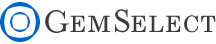X 关闭窗口X 关闭窗口X 关闭窗口大小和重量 Gems are always measured in Millimeter (mm) Dimensions are given as; length x width x depth, except for round stones which are; diameter x depth Select gems by size, not by weight! Gem varieties vary in density, so carat weight is not a good indication of size Note: 1ct = 0.2g